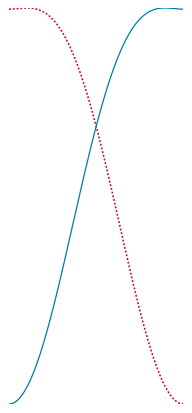Geraint Luff
originalA fun little polynomial cross-fade curve with (almost) constant energy, extended into a family of curves for fractional power-laws.

Last night I was doing some overlap-add processing (for a hacky OLA pitch-shifter), and I needed an energy-preserving cross-fade function.

I played around a bit, and ended up with this:

As you can see, it has a smooth start and end, and a complementary pair of values can be computed quite cheaply:

It's almost (but not exactly) energy-preserving, and has a very slight overshoot.

So why is this interesting, and when might this be useful?

When you're cross-fading two signals, you have to choose whether you want the two curves to sum to a constant amplitude, or a constant energy.

You also commonly want your curves to have a smooth start/end (to minimise clicks), and be cheap to compute - which means if you can avoid sqrt() and sin/cos, that'd be good.

I'm using "amplitude-preserving" here to mean the two curves add up to 1. You generally want this if your signals are correlated, or you are concerned about keeping maximum amplitude (peaks) bounded.

My cheap go-to function for this is the cubic-spline-based curve: x^2(3 - 2x)

The fade-in curve can be computed in 6 operations, and the fade-out curve is just 1 - f(x).

If your inputs are uncorrelated and you're mostly concerned with preserving energy across the fade, the curve above won't add up correctly. Here's the same plot, but with the RMS of the two curves, and you can see that we get a dip in the middle:

The simplest energy-preserving fade to code is probably a cos and sin pair - but they don't taper off nicely around 0:

On top of that, sin and cos are generally expensive to compute. This analysis is a few years old, but estimates sin/cos about 15x slower than add/multiply.

## Approximately energy-preserving

In my particular case (OLA pitch-shifting, which is already a bit approximate), I was willing to accept a small amount of error if there was a speed advantage.

So, I ended up with a pair of polynomials which summed to almost (but not quite) constant energy:

The actual equation is a bit of a mess:

This could be re-arranged to a slightly less strange form - but when you phrase it like this, you can not only compute a value in 7 operations, but (because most of the calculations are shared between fade-in/fade-out) you can get a pair of values in 9 operations.

Here's an example implementation:

I don't really have a good story for how I ended up with this particular solution - I was just messing around with various polynomials, until I stumbled across something good. 😛 The equation above pretty much reflects my route to constructing it.

### Overshoot and RMS error

One odd thing about this curve is that it briefly pops slightly above 1. Here's a zoomed-in version of the previous graph:

It's a relatively small bump (about 0.3%), but unusual enough to be worth mentioning. The RMS only deviates by about ±0.5% (and therefore the energy by ±1%).

You can see the Desmos notebook I made while exploring, including calculating the error statistics for it.

## So...

I found this by just messing about, so there might be an even better one out there.

It's certainly good enough for my use-case, and certainly faster than the code it's replacing. But mostly I just thought it was neat. 😎

## Update: fractional power laws

Firesledge found that replacing our magic constant k = 1.4168 with k = -0.70912 works well for the equal-amplitude case (power-law p=1), with an error of 0.4%.